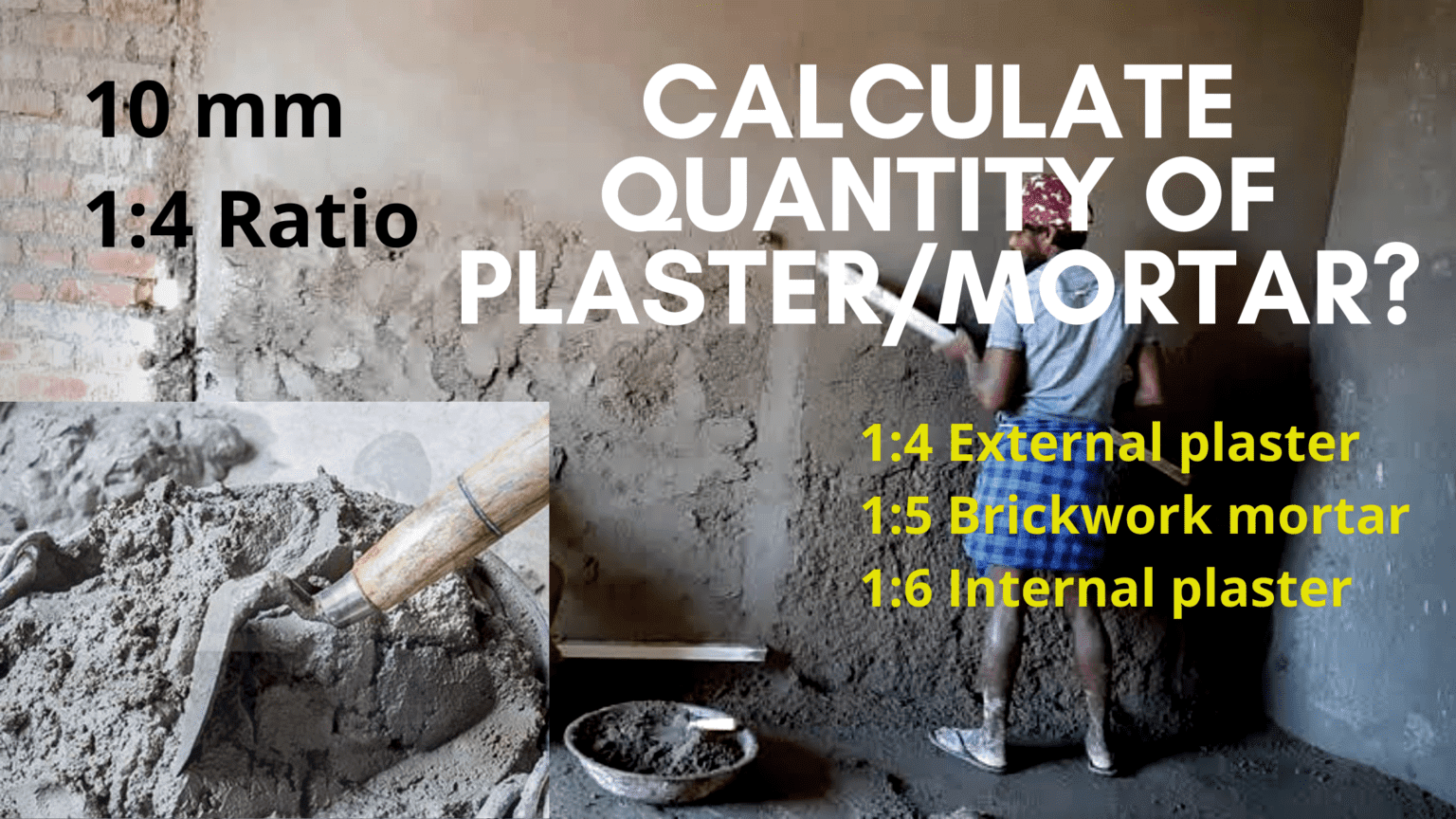# Calculation of plaster: Cement plaster

In this blog, you will learn about calculation of plaster? Further we will calculate quantity of cement and sand in cement-plaster.

## Cement plaster: Difference between mortar and cement?

Mortar is a compound mix of cement & sand or lime & gypsum with water. While the cement is just a bonding agent for cement plaster and mortar. Cement plaster is a same compound mix like mortar, but it has different cement & sand mix ratios. Mortar is used for masonry work, while plaster is used to plastering the walls which is called cement plaster wall. The plaster which is made up with Portland cement is called Portland plaster.

Cement plaster nominal mix ratios are 1:4, 1:5, 1:6, where 1 part of cement and other parts of sand. The plaster mix ratio 1:6 is use for inner walls plastering while 1:4 is use for outer walls plastering. The thickness of plaster is 12mm to 15mm. 1:4 is best cement plaster mix design. visit here

### How to calculate quantity of cement & sand in plaster?

Calculate quantity of cement, sand and water cement ratio in plaster? Formula for calculation of cement sand quantity for plaster mortar. Plaster is the major part of building construction & cement mortar is used for plastring, a little irresponsibility in mortar mixture cost you loss of quality, money.

In this post you will learn about estimation of cement & sand in mortar and water cement ratio. Below we will calculate quantity in Cubic feet as in Cubic Meter.

### How to calculate cement plaster?

How to calculate Area of wall plaster?

1. Area of Wall = L×H
2. Area of Wall = 12’×10′ = 120 sft
`Thickness of plaster = 10mm = 0.375 Inch`

Wet Volume of Mortar = Area × Thickness of plaster

Wet Volume=120×(0.375/12) = 3.75 Cft

• 0.375 divided by 12 to convert inch into foot

Dry Volume of mortar = 3.75×1.33= 4.98 Cft

Or 0.141 Cum

Now we will use dry volume to get Quantity of cement and sand

Ratio of mortar = 1:4

Sum of ratio = 5

### Cement calculation in plaster:

1. Quantity of Cement = (1÷5)×4.98 = 0.996 Cft
2. Cement = (1÷5)×0.141 = 0.0282 Cum

Density of cement = 1440kg/m3 or 40.77kg/cft

Multiply cft with 40.77 & cum with 1440 to get quantity of cement in KGs. Cement consumption in plaster 1:4.

Cft>>>> 0.996×40.77= 40.60 Kg

Cum>>> 0.0282×1440= 40.60 kg

To convert into Bags divide by 50 (50 kg in one bag of cement)

Or directly calculate cement bags from Volume:

Volume of cement bag= 0.034cum or 1.226cft

Cft>>> 0.996÷1.226 = 0.82 Bag

Cum>>> 0.0282÷0.034 = 0.82 Bag

### Sand calculation in plaster:

1. Simply Multiply Dry Volume of cement by 4 (sand is 4 times of cement) OR
2. Sand = (4÷5)×4.98 = 3.984 Cft
3. Quantity of Sand = (4÷5)×0.141 = 0.112 Cum

As Sand is sold & purchased in CFT & CUM, so it don’t need to be converted

If you want to convert it into KGs then multiply Cft Volume with 45.30 and Cum volume with 1600.

Density of sand is 1600kg/m3 OR 45.30 kg/cft

cft>>> 3.984×45.30 = 180.47 kg

Cum>>> 0.112×1600 = 180.47 kg

### Water cement ratio of mortar:

As water is about 0.45 to 0.50 times to cement. To maintain its strength and workability, water should be add with exact ratio. As cement is 40.60 kg so the water will be 20.30 kg/Ltr.

If there is high volume of mortar or concrete, ready mix plaster and ready mix concrete can be used to save time and manpower. How to calculate plastering cost? Just multiply the cement bag with rate of and quantity of sand with rate. Add labour cost for preparation of plaster work.